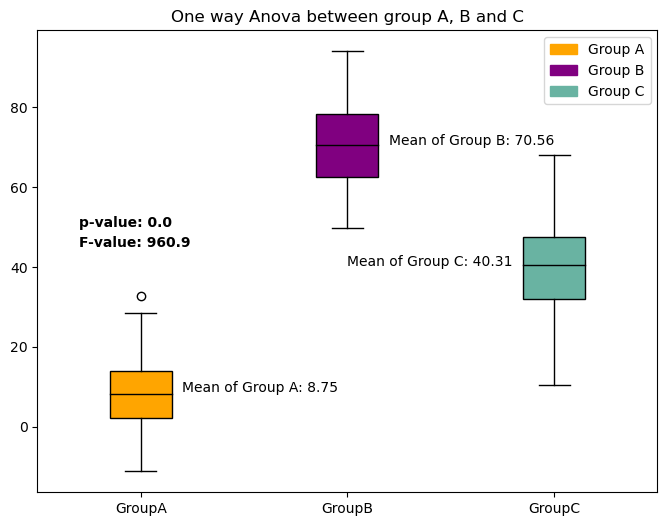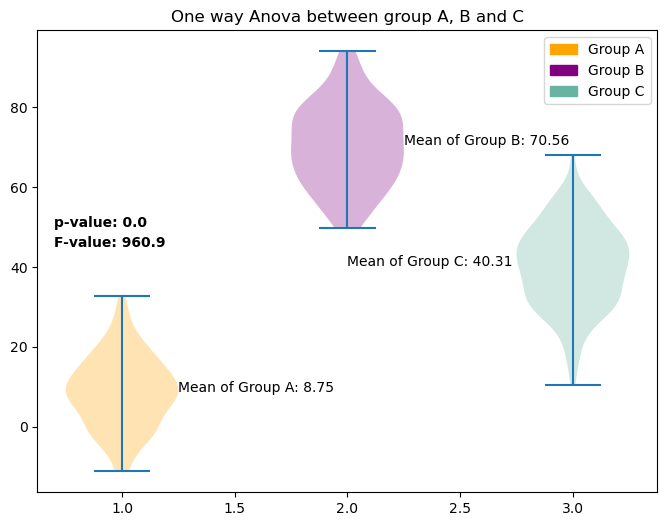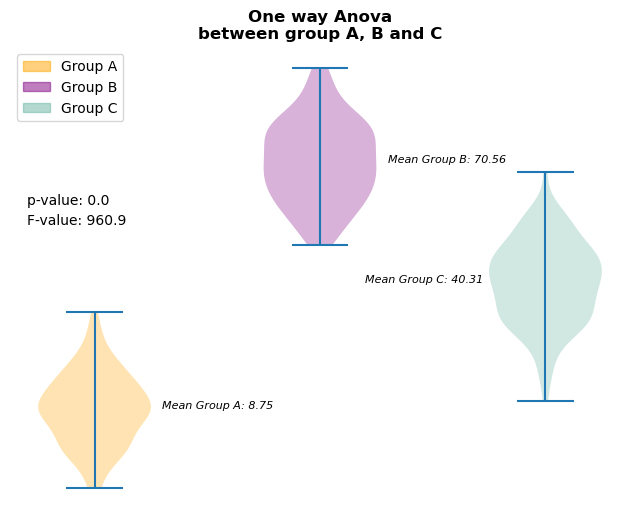The Analysis of Variance (ANOVA) is employed to compare the means of multiple normally distributed variables. Utilizing matplotlib, you can readily generate a plot featuring violin plots or boxplots for these variables on the same chart, enabling a clear depiction of the differences among them.
Furthermore, by employing annotation techniques provided by matplotlib, you can directly incorporate the results of ANOVA into your chart. This enhancement will render the chart more informative and pertinent when comparing distributions among different groups or variables.

## Libraries

First, you need to install the following librairies:

• matplotlib is used for plot creating the charts
• pandas is used to put the data into a dataframe
• `numpy` is used to generate some data

The Anova test will be done using `scipy`: install it using the `pip install scipy` command

``````import pandas as pd
import matplotlib.pyplot as plt
import numpy as np
import scipy.stats as stats``````

## Dataset

Let's create a dummy dataset. Three groups are made up: `A`, `B` and `C`.

For each of them, 100 random values are created thanks to the `np.random.normal()` function, but with different mean values.

``````sample_size = 100

groupA = np.random.normal(10, 10, sample_size)
groupB = np.random.normal(70, 10, sample_size)
groupC = np.random.normal(40, 10, sample_size)

category = ['GroupA']*sample_size + ['GroupB']*sample_size + ['GroupC']*sample_size

df = pd.DataFrame({'value': np.concatenate([groupA, groupB, groupC]),
'category': category})``````

## Get statistical values

First, we'll start by retrieving the values we want to add on the plot: the p value and the F statistic. For this, we need to use the `f_oneway()` function from `scipy`.

Also, we retrieve the mean of each group.

Important: This post does not cover any statistical/math details

``````# groups
groupA = df[df['category']=='GroupA']['value']
groupB = df[df['category']=='GroupB']['value']
groupC = df[df['category']=='GroupC']['value']

# Perform a paired t-test
F_statistic, p_value = stats.f_oneway(groupA, groupB, groupC)

# Get means
mean_groupA = groupA.mean()
mean_groupB = groupB.mean()
mean_groupC = groupC.mean()

# Print the results
print("T-statistic:", F_statistic)
print("P-value:", p_value)
print("Mean groupA:", mean_groupA)
print("Mean groupB:", mean_groupB)
print("Mean groupC:", mean_groupC)``````
```T-statistic: 960.8980055803397 P-value: 2.0225642197230424e-130 Mean groupA: 8.745526783582141 Mean groupB: 70.56101076624377 Mean groupC: 40.310280651985394 ```

Let's round them in order to make the chart more readable at the end

``````F_statistic = round(F_statistic,2)
p_value = round(p_value,5) # more decimal since it's a lower value in general
mean_groupA = round(mean_groupA,2)
mean_groupB = round(mean_groupB,2)
mean_groupC = round(mean_groupC,2)``````

## Boxplot with statistical elements

Now let's use the stats we got above and add them to the plot of boxplots of each group using the `text()` function from matplotlib.

For this graph, we'll also add the average of each group next to its associated boxplot.

``````# Group our dataset with our 'Group' variable
grouped = df.groupby('category')['value']

# Init a figure and axes
fig, ax = plt.subplots(figsize=(8, 6))

# Create the plot with different colors for each group
boxplot = ax.boxplot(x=[group.values for name, group in grouped],
labels=grouped.groups.keys(),
patch_artist=True,
medianprops={'color': 'black'}
)

# Define colors for each group
colors = ['orange', 'purple', '#69b3a2']

# Assign colors to each box in the boxplot
for box, color in zip(boxplot['boxes'], colors):
box.set_facecolor(color)

# Add the p value and the t
p_value_text = f'p-value: {p_value}'
ax.text(0.7, 50, p_value_text, weight='bold')
f_value_text = f'F-value: {F_statistic}'
ax.text(0.7, 45, f_value_text, weight='bold')

# Add the mean for each group
ax.text(1.2, mean_groupA, f'Mean of Group A: {mean_groupA}', fontsize=10)
ax.text(2.2, mean_groupB, f'Mean of Group B: {mean_groupB}', fontsize=10)
ax.text(2, mean_groupC, f'Mean of Group C: {mean_groupC}', fontsize=10)

# Add a title and axis label
ax.set_title('One way Anova between group A, B and C')

legend_labels = ['Group A', 'Group B', 'Group C']
legend_handles = [plt.Rectangle((0,0),1,1, color=color) for color in colors]
ax.legend(legend_handles, legend_labels)

# Display it
plt.show()``````## Violin plot with statistical elements

``````# Group our dataset with our 'Group' variable
grouped = df.groupby('category')['value']

# Init a figure and axes
fig, ax = plt.subplots(figsize=(8, 6))

# Create the plot with different colors for each group
violins = ax.violinplot([group.values for name, group in grouped],
#labels=grouped.groups.keys()
)

# Define colors for each group
colors = ['orange', 'purple', '#69b3a2']

# Assign colors to each box in the boxplot
for violin, color in zip(violins['bodies'], colors):
violin.set_facecolor(color)

# Add the p value and the t
p_value_text = f'p-value: {p_value}'
ax.text(0.7, 50, p_value_text, weight='bold')
F_value_text = f'F-value: {F_statistic}'
ax.text(0.7, 45, F_value_text, weight='bold')

# Add the mean for each group
ax.text(1.25, mean_groupA, f'Mean of Group A: {mean_groupA}', fontsize=10)
ax.text(2.25, mean_groupB, f'Mean of Group B: {mean_groupB}', fontsize=10)
ax.text(2, mean_groupC, f'Mean of Group C: {mean_groupC}', fontsize=10)

# Add a title and axis label
ax.set_title('One way Anova between group A, B and C')

legend_labels = ['Group A', 'Group B', 'Group C']
legend_handles = [plt.Rectangle((0,0),1,1, color=color) for color in colors]
ax.legend(legend_handles, legend_labels)

# Display it
plt.show()``````## Customized violin plot with statistics

``````# Group our dataset with our 'Group' variable
grouped = df.groupby('category')['value']

# Init a figure and axes
fig, ax = plt.subplots(figsize=(8, 6))

# Create the plot with different colors for each group
violins = ax.violinplot([group.values for name, group in grouped],
#labels=grouped.groups.keys()
)

# Define colors for each group
colors = ['orange', 'purple', '#69b3a2']

# Assign colors to each box in the boxplot
for violin, color in zip(violins['bodies'], colors):
violin.set_facecolor(color)

# Add the p value and the t
p_value_text = f'p-value: {p_value}'
ax.text(0.7, 60, p_value_text)
F_value_text = f'F-value: {F_statistic}'
ax.text(0.7, 55, F_value_text)

# Add the mean for each group
ax.text(1.3, mean_groupA, f'Mean Group A: {mean_groupA}',
style='italic', fontsize=8)
ax.text(2.3, mean_groupB, f'Mean Group B: {mean_groupB}',
style='italic', fontsize=8)
ax.text(2.2, mean_groupC, f'Mean Group C: {mean_groupC}',
style='italic', fontsize=8)

# Remove axis labels
ax.set_xticks([])
ax.set_yticks([])

# Removes spines
ax.spines[['right', 'top', 'left', 'bottom']].set_visible(False)

# Add a title and axis label
ax.set_title('One way Anova\nbetween group A, B and C', weight='bold')

legend_labels = ['Group A', 'Group B', 'Group C']
legend_handles = [plt.Rectangle((0,0),1,1, color=color, alpha=0.5) for color in colors]
ax.legend(legend_handles, legend_labels, loc='upper left')

# Display it
plt.show()``````## Going further

This post explains how to represent the results of an Anova in a violin plot and a boxplot.

For more examples of charts with statistics, see the statistics section. You may also be interested in how to represent Student t-test results.

## Contact & Edit

👋 This document is a work by Yan Holtz. You can contribute on github, send me a feedback on twitter or subscribe to the newsletter to know when new examples are published! 🔥